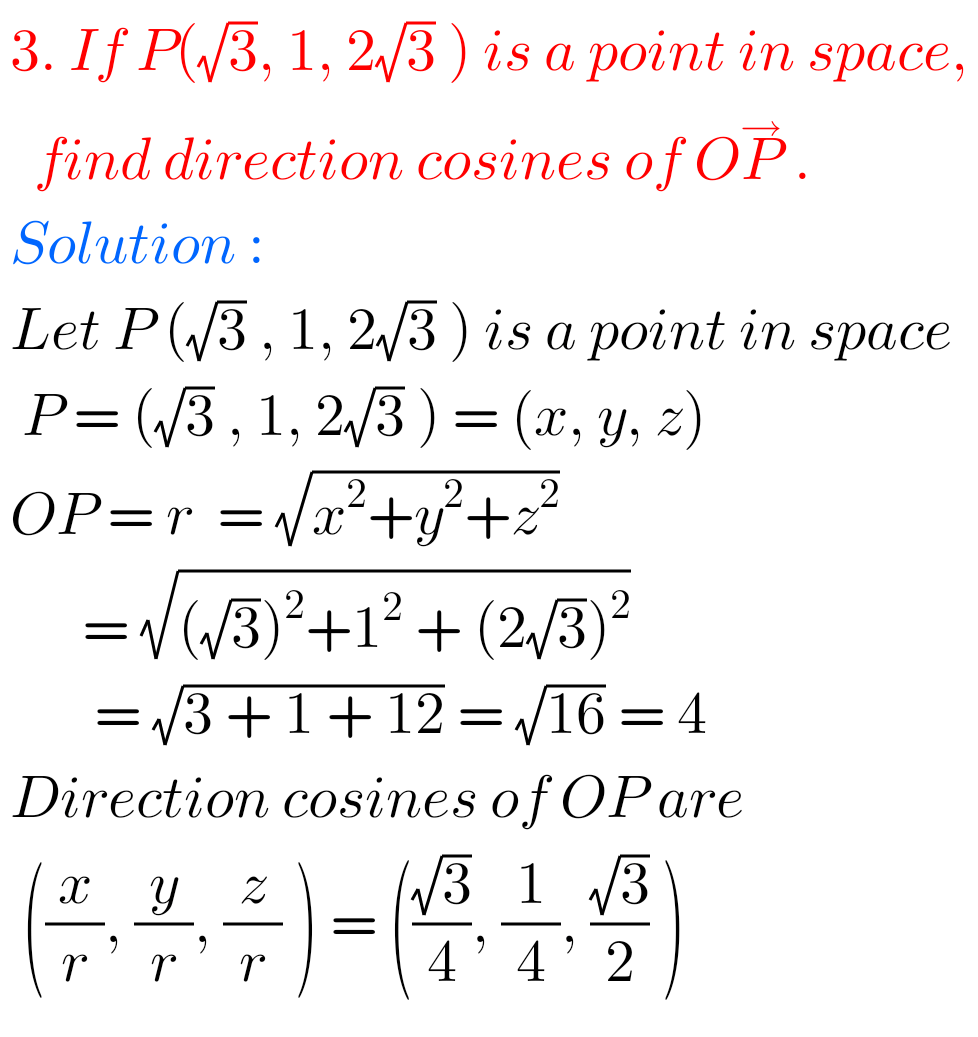## Inter Maths 1B Solutions for Direction Cosines and Direction Ratios Exercise 6(a)

Intermediate Maths 1B Solutions for Direction Cosines and Direction Ratios Exercise 6(a) Solutions for textbook Exercise 6(a) Direction Cosines and Direction Ratios Maths 1B Intermediate Mathematics. Study the textbook lesson very well. Then Observe the example problems and solutions given in the textbook. These solutions are easy to understand. Observe the given solutions and try …